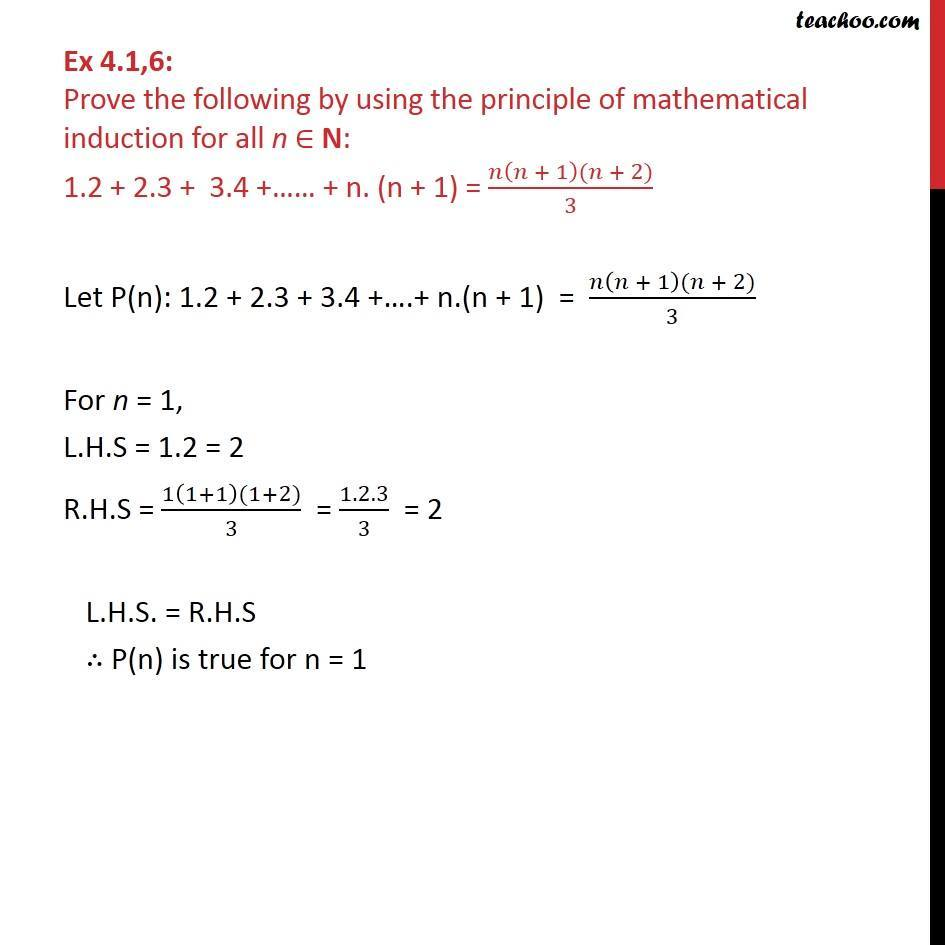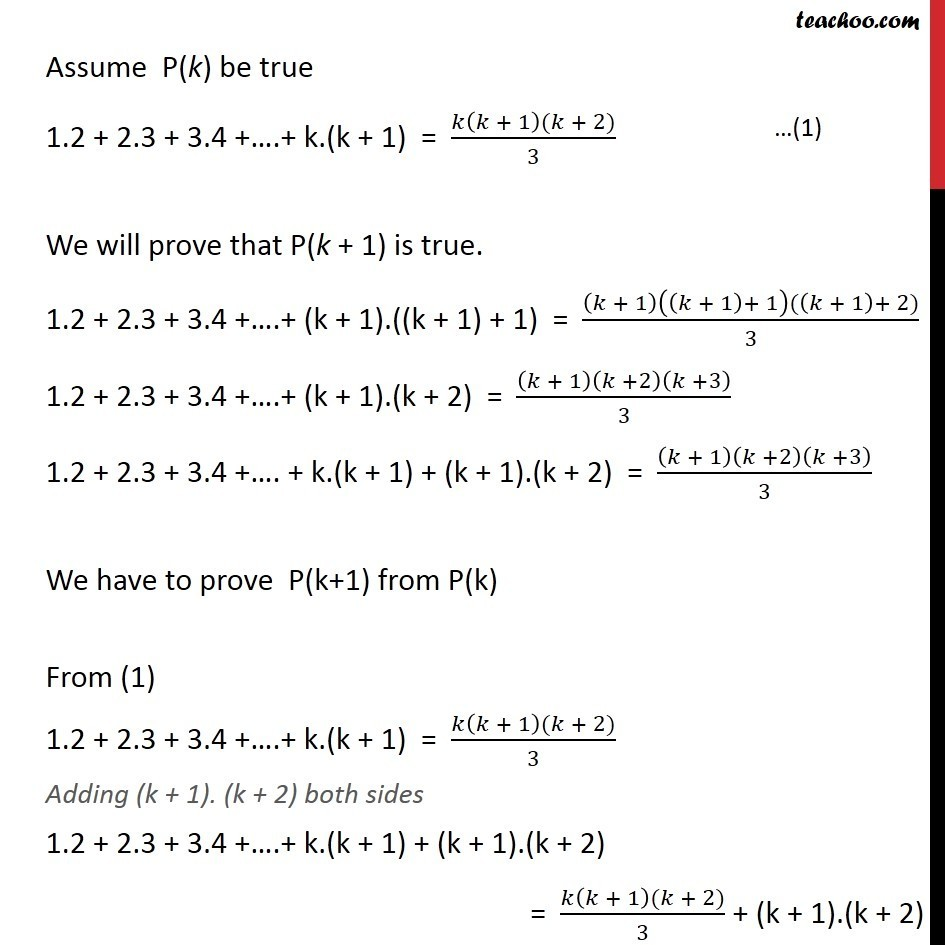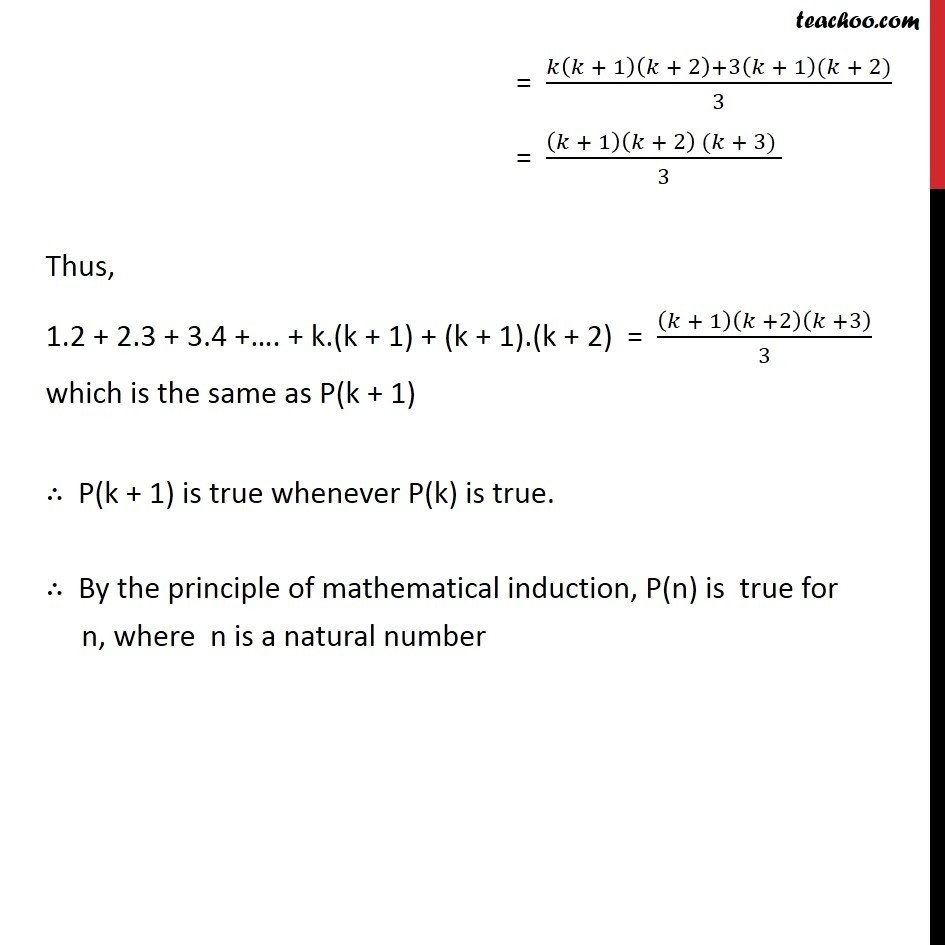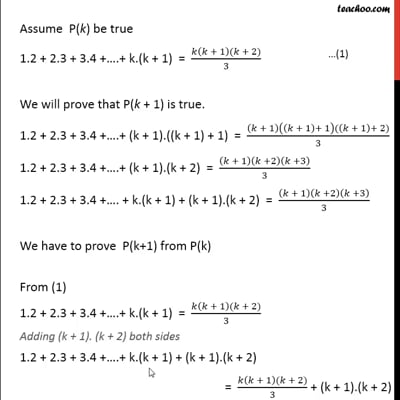Chapter 4 Class 11 Mathematical Induction
Concept wiseThis video is only available for Teachoo black users

Introducing your new favourite teacher - Teachoo Black, at only ₹83 per month

### Transcript

Ex 4.1,6: Prove the following by using the principle of mathematical induction for all n ∈ N: 1.2 + 2.3 + 3.4 +…… + n. (n + 1) = (𝑛(𝑛 + 1)(𝑛 + 2))/3 Let P(n): 1.2 + 2.3 + 3.4 +….+ n.(n + 1) = (𝑛(𝑛 + 1)(𝑛 + 2))/3 For n = 1, L.H.S = 1.2 = 2 R.H.S = (1(1+1)(1+2))/3 = 1.2.3/3 = 2 L.H.S. = R.H.S ∴ P(n) is true for n = 1 Assume P(k) be true 1.2 + 2.3 + 3.4 +….+ k.(k + 1) = (𝑘(𝑘 + 1)(𝑘 + 2))/3 We will prove that P(k + 1) is true. 1.2 + 2.3 + 3.4 +….+ (k + 1).((k + 1) + 1) = ((𝑘 + 1)((𝑘 + 1)+ 1)((𝑘 + 1)+ 2))/3 1.2 + 2.3 + 3.4 +….+ (k + 1).(k + 2) = (𝑘 + 1)(𝑘 +2)(𝑘 +3)/3 1.2 + 2.3 + 3.4 +…. + k.(k + 1) + (k + 1).(k + 2) = (𝑘 + 1)(𝑘 +2)(𝑘 +3)/3 We have to prove P(k+1) from P(k) From (1) 1.2 + 2.3 + 3.4 +….+ k.(k + 1) = (𝑘(𝑘 + 1)(𝑘 + 2))/3 Adding (k + 1). (k + 2) both sides 1.2 + 2.3 + 3.4 +….+ k.(k + 1) + (k + 1).(k + 2) = (𝑘(𝑘 + 1)(𝑘 + 2))/3 + (k + 1).(k + 2) = (𝑘(𝑘 + 1)(𝑘 + 2)+3(𝑘 + 1)(𝑘 + 2))/3 = ((𝑘 + 1)(𝑘 + 2) (𝑘 + 3) )/3 Thus, 1.2 + 2.3 + 3.4 +…. + k.(k + 1) + (k + 1).(k + 2) = (𝑘 + 1)(𝑘 +2)(𝑘 +3)/3 which is the same as P(k + 1) ∴ P(k + 1) is true whenever P(k) is true. ∴ By the principle of mathematical induction, P(n) is true for n, where n is a natural number